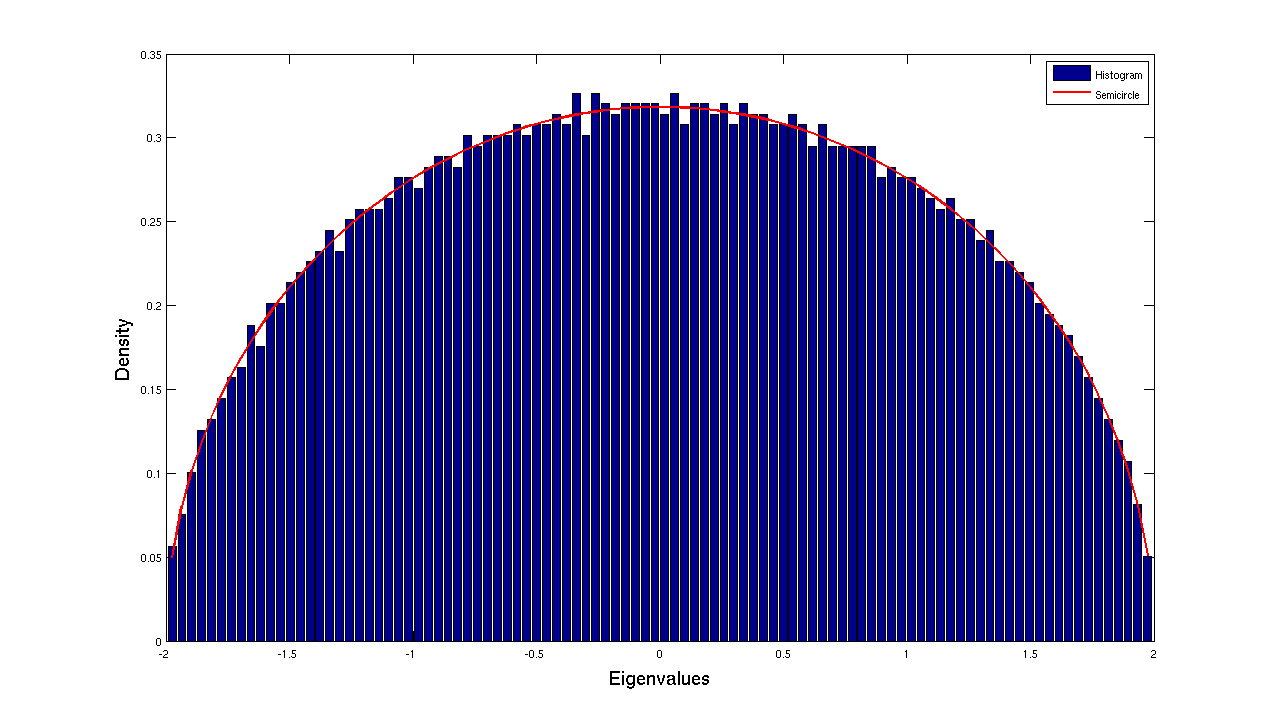I did my PhD on Spectrum of Random Band Matrices under the supervision of professor Alexander Soshnikov. My MS thesis was on matchings between Point processes .

## Publications and Preprints

1. [Abstract] Circular Law for Random Block Band Matrices with Genuinely Sublinear Bandwidth (with Vishesh Jain, Kyle Luh, Sean O'Rourke),
Journal of Mathematical Physics, Volume 62, Issue 8
We prove the circular law for a class of non-Hermitian random block band matrices with genuinely sublinear bandwidth. Namely, we show there exists $\tau \in (0,1)$ so that if the bandwidth of the matrix $X$ is at least $n^{1-\tau}$ and the nonzero entries are iid random variables with mean zero and slightly more than four finite moments, then the limiting empirical eigenvalue distribution of $X$, when properly normalized, converges in probability to the uniform distribution on the unit disk in the complex plane. The key technical result is a least singular value bound for shifted random band block matrices with genuinely sublinear bandwidth, which improves on a result of Cook in the band matrix setting \cite{cook2018lower}.
2. [Abstract] CLT for non-Hermitian random band matrices with variance profiles
arXiv preprint: arXiv: 1904.11098, 2019
We show that the fluctuations of the linear eigenvalue statistics of a non-Hermitian random band matrix of bandwidth $b_{n}$ with a continuous variance profile $w_{\nu}(x)$ converges to a $N(0,\sigma_{f}^{2}(\nu))$, where $\nu=\lim_{n\to\infty}(2b_{n}/n)$. We obtain an explicit formula for $\sigma_{f}^{2}(\nu)$, which depends on the test function, and $w_{\nu}$. When $\nu=1$, the formula is consistent with Rider, and Silverstein (2006). We also compute an explicit formula for $\sigma_{f}^{2}(0)$. We show that $\sigma_{f}^{2}(\nu)\to \sigma_{f}^{2}(0)$ as $\nu\downarrow 0$.
3. [Abstract] Linear eigenvalue statistics of random matrices with a variance profile, (with Kartick Adhikari, Koushik Saha)
Random Matrices: Theory and Applications
We give an upper bound on the total variation distance between the linear eigenvalue statistic, properly scaled and centred, of a random matrix with a variance profile and the standard Gaussian random variable. The second order Poincaré inequality type result is used to establish the bound. Using this bound we prove Central limit theorem for linear eigenvalue statistics of random matrices with different kind of variance profiles. We re-establish some existing results on fluctuations of linear eigenvalue statistics of some well known random matrix ensembles by choosing appropriate variance profiles.
4. [Abstract] Distribution of singular values of random band matrices; Marchenko-Pastur law and more, (with Alexander Soshnikov)
Journal of Statistical Physics, 168(5), 964-985, doi:10.1007/s10955-017-1844-5
We consider the limiting spectral distribution of matrices of the form $\frac{1}{2b_{n}+1} (R + X)(R + X)^{*}$, where $X$ is an $n\times n$ band matrix of bandwidth $b_{n}$ and $R$ is a non random band matrix of bandwidth $b_{n}$. We show that the Stieltjes transform of ESD of such matrices converges to the Stieltjes transform of a non-random measure. And the limiting Stieltjes transform satisfies an integral equation. For $R=0$, the integral equation yields the Stieltjes transform of the Marchenko-Pastur law.
5. [Abstract] Fluctuations of Linear Eigenvalue Statistics of Random band Matrices, (with Koushik Saha, Alexander Soshnikov)
Theory of Probablity and Applications, Vol 60, No. 3, 407--443, 2016.
In this paper, we study the fluctuation of linear eigenvalue statistics of Random Band Matrices defined by $M_{n}=\frac{1}{\sqrt{b_{n}}}W_{n}$, where $W_{n}$ is a $n\times n$ band Hermitian random matrix of bandwidth $b_{n}$, i.e., the diagonal elements and only first $b_{n}$ off diagonal elements are nonzero. Also variances of the matrix elmements are upto a order of constant. We study the linear eigenvalue statistics $\mathcal{N}(\phi)=\sum_{i=1}^{n}\phi(\lambda_{i})$ of such matrices, where $\lambda_{i}$ are the eigenvalues of $M_{n}$ and $\phi$ is a sufficiently smooth function. We prove that $\sqrt{\frac{b_{n}}{n}}[\mathcal{N}(\phi)-\mathbb{E} \mathcal{N}(\phi)]\stackrel{d}{\to} N(0,V(\phi))$ for $b_{n}>>\sqrt{n}$, where $V(\phi)$ is given in the Theorem 1.